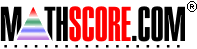Math Practice Online > free > lessons > Florida > 6th grade > Fractions to Decimals

## Fractions to Decimals

Convert fractions to decimals using denominators that divide evenly into 100. You may need pencil and paper.

 Sample Problems for Fractions to Decimals Lesson for Fractions to Decimals

### This topic aligns to the following state standards

Grade 3: Num 2. Identifies equivalent forms of numbers.
Grade 3: Num 3. Knows that two numbers in different forms are equivalent or non-equivalent, using whole numbers, fractions, and decimals in the context of money.
Grade 4: Num 2. Identifies equivalent forms of numbers.
Grade 4: Num 3. Knows that two numbers in different forms are equivalent or non-equivalent, using whole numbers, decimals, fractions, and mixed numbers.
Grade 5: Num 1. Knows that numbers in different forms are equivalent or nonequivalent, using whole numbers, decimals, fractions, mixed numbers, and percents.
Grade 6: Num 4. Converts a number expressed in one form to its equivalent in another form.
Grade 7: Num 4. Converts a number expressed in one form to its equivalent in another form.
Grade 9: Num 4. understands that numbers can be represented in a variety of equivalent forms, including integers, fractions, decimals, percents, scientific notation, exponents, radicals, absolute value, and logarithms

Copyright Accurate Learning Systems Corporation 2008.